Math resources Geometry Angles

Complementary angles

# Complementary angles

Here you will learn about complementary angles, including how to find missing angles by applying knowledge of complementary angles.

Students will first learn about complementary angles as a part of measurement and data in 4th grade. They will expand that knowledge as they progress through middle school.

## What are complementary angles?

Complementary angles are two angles that add up to 90 degrees. They can be adjacent or not adjacent. Adjacent angles are angles that share a common arm and a vertex.

Adjacent complementary angles make a right angle.

You can use what you know about complementary angles to decompose angles and find the measurement of an unknown angle.

### What are complementary angles?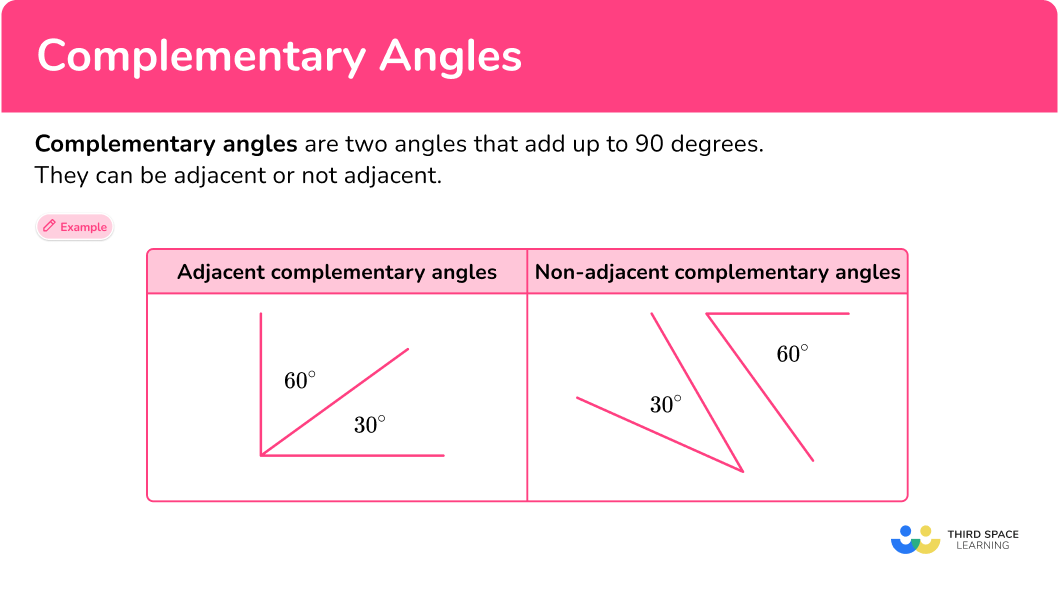## Common Core State Standards

How does this relate to 4th grade math?

• Grade 4: Measurement and Data (4.MD.C.7)
Recognize angle measure as additive. When an angle is decomposed into non-overlapping parts, the angle measure of the whole is the sum of the angle measures of the parts.

Solve addition and subtraction problems to find unknown angles on a diagram in real world and mathematical problems, for example, by using an equation with a symbol for the unknown angle measure.

## How to find the measure of complementary angles

In order to find the measure of complementary angles you need to:

1. Determine which angles are complementary.
2. Identify given angle measurements and the unknown angle.
3. Find the missing angle.

## Complementary angles examples

### Example 1: finding complementary angles (adjacent angles)

The two angles shown, x and y, are complementary. Find the measure of angle x.

1. Determine which angles are complementary.

The question states that angles x and y are complementary and equal 90^{\circ}.

x+y=90

2Identify given angle measurements and the unknown angle or angles.

Angle y is 35^{\circ}.

The unknown angle is angle x, and when it’s added to 35^{\circ} will equal 90^{\circ}.

x+35=90

3Find the missing angle.

To find the missing angle, you will subtract 35 from 90.

x=90-35

x =55

The measurement of angle x is 55^{\circ}.

### Example 2: finding complementary angles (adjacent angles)

The two angles shown are complementary. Find the measure of angle x.

The question states that angles x and y are complementary and equal 90^{\circ}.

x+y=90

Angle y is 62^{\circ}.

The unknown angle is angle x, and when it’s added to 62^{\circ} will equal 90^{\circ}.

x+62=90

To find the missing angle, you will subtract 62 from 90.

x=90-62

x =28

The measurement of angle x is 28^{\circ}.

### Example 3: finding complementary angles (not adjacent angles)

The two angles shown are complementary. Find the measure of angle a.

The question states that angles a and b are complementary and equal 90^{\circ}.

a+b=90

Angle b is 59^{\circ}.

The unknown angle is angle a, and when it’s added to 59^{\circ} will equal 90^{\circ}.

a+59=90

To find the missing angle, you will subtract 59 from 90.

a=90-59

a =31

The measurement of angle a is 31^{\circ}.

### Example 4: finding complementary angles (not adjacent angles)

The two angles shown are complementary. Find the measure of angle c.

The question states that angles b and c are complementary and equal 90^{\circ}.

b+c=90

Angle b is 43^{\circ}.

The unknown angle is angle c, and when it’s added to 43^{\circ} will equal 90^{\circ}.

43+c=90

To find the missing angle, you will subtract 43 from 90.

c=90-43

c =47

The measurement of angle c is 47^{\circ}.

### Example 5: finding an angle which is complementary to another

Two angles ‘x and y' are complementary and one of them is 49^{\circ}. What is the size of the other angle?

The two angles are complementary and therefore equal 90^{\circ}.

x+y=90

Because it is not stated which angle is 49^{\circ}, you can assume that \angle x has a measure of 49^{\circ}.

49^{\circ}+y=90^{\circ}

You will subtract 49 from 90 to find the measure of the missing angle.

y=90-49

y=41^{\circ}

The size of the other angle is 41^{\circ}.

### Example 6: finding an angle which is complementary to another

Two angles ‘x and y' are complementary and one of them is 17^{\circ}. What is the size of the other angle?

The two angles are complementary and therefore equal 90^{\circ}.

x+y=90

Because it is not stated which angle is 17^{\circ}, you can assume that \angle x has a measure of 17^{\circ}.

17^{\circ}+y=90^{\circ}

You will subtract 17 from 90 to find the measure of the missing angle.

y=90-17

y=73^{\circ}

The size of the other angle is 73^{\circ}.

### Teaching tips for complementary angles

• Rather than having students practice finding and decomposing complementary angles on multiple skill worksheets, provide them with a variety of practice problems, activities, and/or projects that have a real-world context. This will deepen their understanding of this skill.

### Easy mistakes to make

• Mixing up supplementary angles and complementary angles
Students may mix up supplementary and complementary angles, thinking that complementary angles add up to 180^{\circ} and that supplementary angles add up to 90^{\circ}. However, complementary angles add up to 90^{\circ} and supplementary angles add up to 180^{\circ}.

• Assuming complementary angles always have a common vertex
Complementary angles can be either adjacent or not adjacent. Not adjacent complementary angles do not share a common vertex, but their angles will still add up to 180^{\circ}.

### Complementary angles practice questions

1. \angle l and \angle m are complementary angles. \angle l measures at 19^{\circ}. What is the measure of \angle m?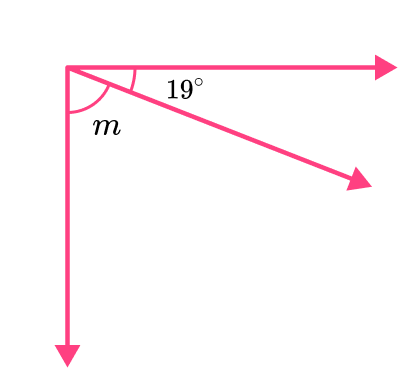m=61^{\circ}m=161^{\circ}m=154^{\circ}m=71^{\circ}The two angles are complementary, so they must have a sum of 90.

You will subtract 19 from 90 to find the measure of the missing angle.

m=90-19

m=71

The missing angle measures at 71^{\circ}.

2. \angle j and \angle k are complementary angles. \angle j measures at 45^{\circ}. What is the measure of \angle k?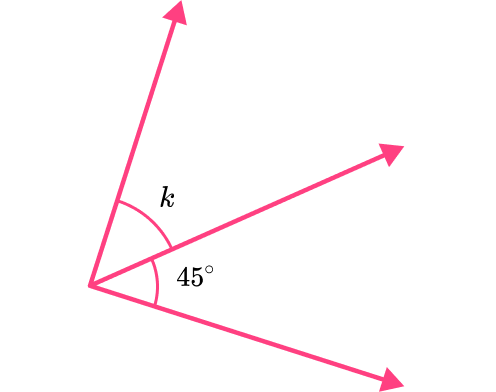k=43^{\circ}k=45^{\circ}k=145^{\circ}k=143^{\circ}The two angles are complementary, so they must have a sum of 90.

You will subtract 45 from 90 to find the measure of the missing angle.

k=90-45

k=45

The missing angle measures at 45^{\circ}.

3. \angle w and \angle z are complementary angles. \angle z measures at 36^{\circ}. What is the measure of \angle w?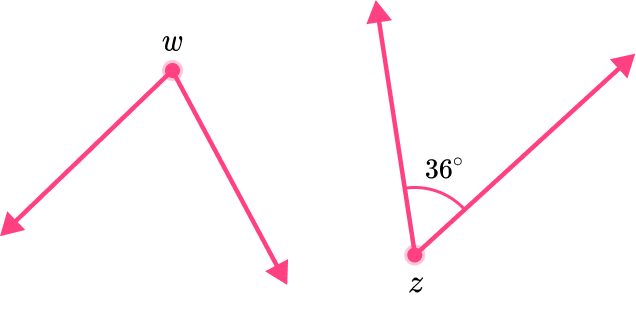w=154^{\circ}w=47^{\circ}w=54^{\circ}w=132^{\circ}The two angles are complementary, so they must have a sum of 90.

You will subtract 36 from 90 to find the measure of the missing angle.

w=90-36

w=54

The missing angle measures at 54^{\circ}.

4. \angle f and \angle e are complementary angles. \angle f measures at 66^{\circ}. What is the measure of \angle e?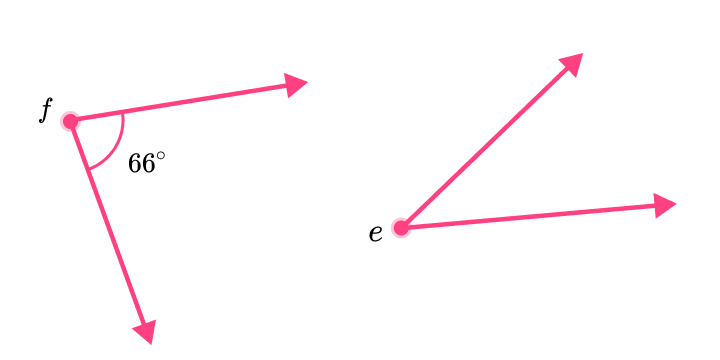e=123^{\circ}e=90^{\circ}e=44^{\circ}e=24^{\circ}The two angles are complementary, so they must have a sum of 90.

You will subtract 66 from 90 to find the measure of the missing angle.

e=90-66

e=24

The missing angle measures at 24^{\circ}.

5. Two angles ‘x and y' are complementary and one of them is 47^{\circ}. What is the size of the other angle?

x=33^{\circ}x=43^{\circ}x=133^{\circ}x=313^{\circ}The two angles are complementary, so they must have a sum of 90.

You will subtract 47 from 90 to find the measure of the missing angle.

x=90-47

x=43

The missing angle measures at 43^{\circ}.

6. Two angles ‘x and y' are complementary and one of them is 3^{\circ}. What is the size of the other angle?

x=87^{\circ}x=3^{\circ}x=93^{\circ}x=177^{\circ}The two angles are complementary, so they must have a sum of 90.

You will subtract 3 from 90 to find the measure of the missing angle.

x=90-3

x=87

The missing angle measures at 87^{\circ}.

## Complementary angles FAQs

What is the definition of complementary angles?

What’s the difference between complementary and supplementary angles?

Complementary angles add up to 90 degrees, or a right angle.
Supplementary angles add up to 180 degrees, or a straight line or straight angle.

Can three angles be complementary?

No, three angles will never be complementary, even if the sum of their measurement is 90^{\circ}. You will only see a pair of complementary angles.

Is there a formula for complementary angles?

\angle a+ \angle b=90^{\circ}; two angles are complementary if angle \, a and angle \, b equal 90^{\circ}.

## Still stuck?

At Third Space Learning, we specialize in helping teachers and school leaders to provide personalized math support for more of their students through high-quality, online one-on-one math tutoring delivered by subject experts.

Each week, our tutors support thousands of students who are at risk of not meeting their grade-level expectations, and help accelerate their progress and boost their confidence.